# Polynomial FactorizationPage 1

#### WATCH ALL SLIDES

Slide 1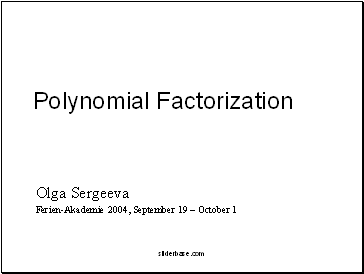Polynomial Factorization

Olga Sergeeva

Ferien-Akademie 2004, September 19 – October 1

Slide 2Overview

Univariate Factorization

Overview of the algoriths and the required simplifications

Factoring over finite fields

Factorization based on Hensel lifting

LLL algorith

Multivariate Factorization

Problems overview

The idea of the algorith

Analysis of correctness probability.

Slide 3## Univariate Factorization – algoriths

We consider factorization of polynomials over the rational integers, Z, and different approaches to this problem.

Slide 4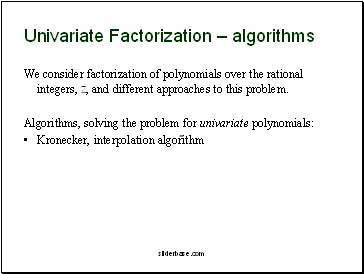Univariate Factorization – algoriths

We consider factorization of polynomials over the rational integers, Z, and different approaches to this problem.

Algoriths, solving the problem for univariate polynomials:

Kronecker, interpolation algorith

Slide 5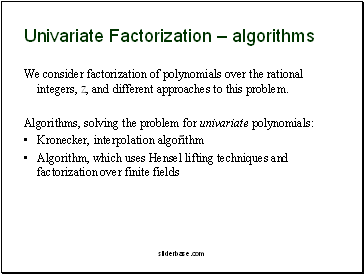Univariate Factorization – algoriths

We consider factorization of polynomials over the rational integers, Z, and different approaches to this problem.

Algoriths, solving the problem for univariate polynomials:

Kronecker, interpolation algorith

Algorith, which uses Hensel lifting techniques and factorization over finite fields

Slide 6Univariate Factorization – algoriths

We consider factorization of polynomials over the rational integers, Z, and different approaches to this problem.

Algoriths, solving the problem for univariate polynomials:

Kronecker, interpolation algorith

Algorith, which uses Hensel lifting techniques and factorization over finite fields

A. K. Lenstra, H. W. Lenstra and Lovasz – polynomial time algorith using basic reduction techniques for lattices.

Slide 7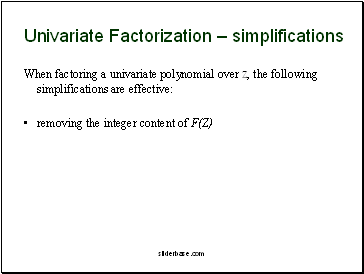## Univariate Factorization – simplifications

When factoring a univariate polynomial over Z, the following simplifications are effective:

removing the integer content of F(Z)

Slide 8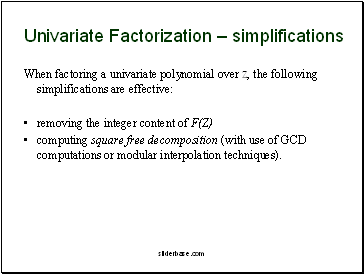Univariate Factorization – simplifications

When factoring a univariate polynomial over Z, the following simplifications are effective:

removing the integer content of F(Z)

computing square free decomposition (with use of GCD computations or modular interpolation techniques).

Slide 9Go to page:
1  2  3  4  5  6  7